#### Number of problems found: 276

• Find the 20Find the product and the sum of the roots of x2 + 3x - 9 = 0
• Area and perimeter of rectangleThe content area of the rectangle is 3000 cm2, one dimension is 10 cm larger than the other. Determine the perimeter of the rectangle.
• Integer sidesA right triangle with an integer length of two sides has one leg √11 long. How much is its longest side?
• Perimeter and diagonal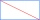The perimeter of the rectangle is 82 m, the length of its diagonal is 29 m. Find the dimensions of the rectangle.
• On a lineOn a line p : 3 x - 4 y - 3 = 0, determine the point C equidistant from points A[4, 4] and B[7, 1].
• Function 3Function f(x)=a(x-r)(x-s) the graph of the function has x- intercept at (-4, 0) and (2, 0) and passes through the point (-2,-8). Find constant a, r, s.
• StaircaseOn a staircase 3.6 meters high, the number of steps would increase by 3 if the height of one step decreased by 4 cm. How high are the stairs?
• Lookout towerHow high is the lookout tower? If each step was 3 cm lower, there would be 60 more of them on the lookout tower. If it was 3 cm higher again, it would be 40 less than it is now.
• Pagans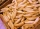Jano and Michael ate pagans. Jano ate three more than Michael. The product of their counts (numbers) is 180. How many pagans did each of them eat?
• The cylinderThe cylinder has a surface area of 300 square meters, while the height of the cylinder is 12 m. Calculate the volume of this cylinder.
• 1 page1 page is torn from the book. The sum of the page numbers of all the remaining pages is 15,000. What numbers did the pages have on the page that was torn from the book?
• BirthdaysIn the classroom, students always give candy to their classmates on their birthdays. The birthday person always gives each one candy, and he does not give himself. A total of 650 candies were distributed in the class per year. How many students are in the
• Dimensions of the trapezoidOne of the bases of the trapezoid is one-fifth larger than its height, the second base is 1 cm larger than its height. Find the dimensions of the trapezoid if its area is 115 cm2
• Isosceles triangleIn an isosceles triangle ABC with base AB; A [3,4]; B [1,6] and the vertex C lies on the line 5x - 6y - 16 = 0. Calculate the coordinates of vertex C.
• Two grovesTwo groves A, B are separated by a forest, both are visible from the hunting grove C, which is connected to both by direct roads. What will be the length of the projected road from A to B, if AC = 5004 m, BC = 2600 m and angle ABC = 53° 45 ’?
• Roots and coefficient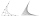In the equation 2x ^ 2 + bx-9 = 0 is one root x1 = -3/2. Determine the second root and the coefficient b.
• Points in space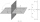There are n points, of which no three lie on one line and no four lies on one plane. How many planes can be guided by these points? How many planes are there if there are five times more than the given points?
• Find the 15Find the tangent line of the ellipse 9 x2 + 16 y2 = 144 that has the slope k = -1
• Tangents to ellipseFind the magnitude of the angle at which the ellipse x2 + 5 y2 = 5 is visible from the point P[5, 1] .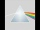Please determine the solvability conditions of the equation, solve the equation and perform the test: x divided by x squared minus 2x plus1 the whole minus x + 3 divided by x squared minus 1 this is equal to 0: x/(x2-2x+1) - (x+3)/( x2-1) = 0